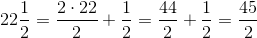## Example Questions

### Example Question #1 : How To Find The Reciprocal Of A Fraction

Carpenter A takes 45 hours to build a table. Carpenter B takes 30 hours. When they work together, how long will it take them to build a table?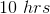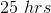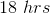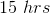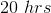Explanation:

Write their respective rates as fractions:

Carpenter A: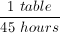Carpenter B: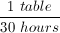Add them together to find their combined rate.  First find a common denominator.  The smallest multiple of both 45 and 30 is 90: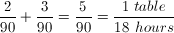Therefore, they take 18 hours to build a table together.

### Example Question #2 : How To Find The Reciprocal Of A Fraction

What is the sum of the reciprocal of, the reciprocal of, the reciprocal of, and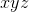?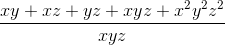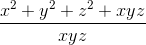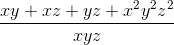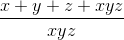Explanation:

Begin by finding the reciprocal of, the reciprocal of, and the reciprocal of: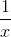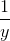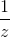In order to add the four terms together, we will need to find a common denominator. Find the common denominator by multiplying the three different terms in the denominator (x, y, and z). Our common denominator will be. Put each term into terms of this common denominator: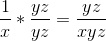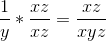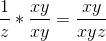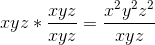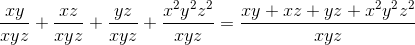Look for the answer choice that matches this.

### Example Question #3 : How To Find The Reciprocal Of A Fraction

Find the reciprocal of the following expression: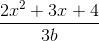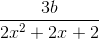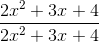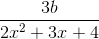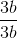Explanation:

To find the reciprocal of a rational expression--any rational expression--all you need to do is flip the numerator and the denominator.You can check this by multiplying the two expressions together and ensuring that your answer is.

### Example Question #4 : How To Find The Reciprocal Of A Fraction

Find the reciprocal of the following expression: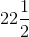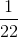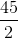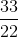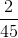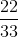Explanation:

To find the reciprocal of any fraction, you merely need to invert the numerator and denominator.

In this case, first you have to convert the mixed number into a fraction: